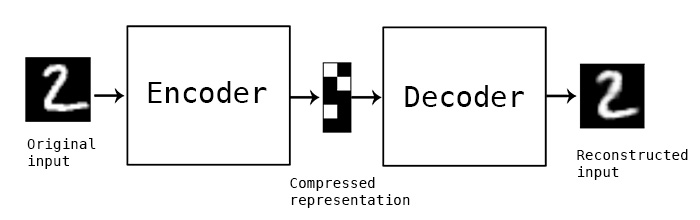In [ ]: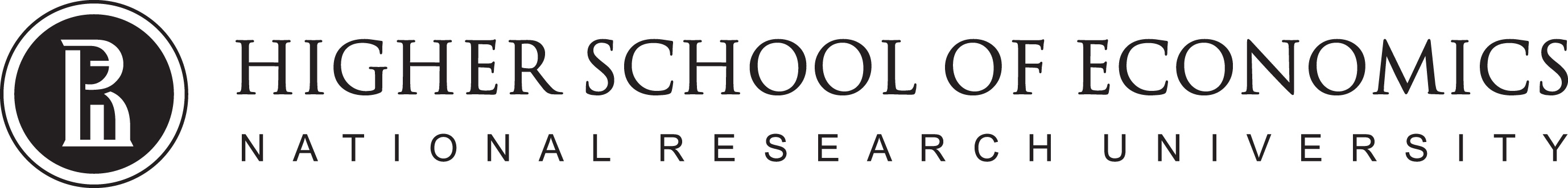# Data Analysis

## Neural Networks 21

1. Some materials are taken from machine learning course of Victor Kitov

# Let's recall previous lecture¶

## Feed-Forward Nets¶

• NNs have started long time ago
• Composed of layers
• Layers composed of neurons
• Each layers sum up outputs of previous layer and put it throught some non-linear activation function
• Backpropagation algorithm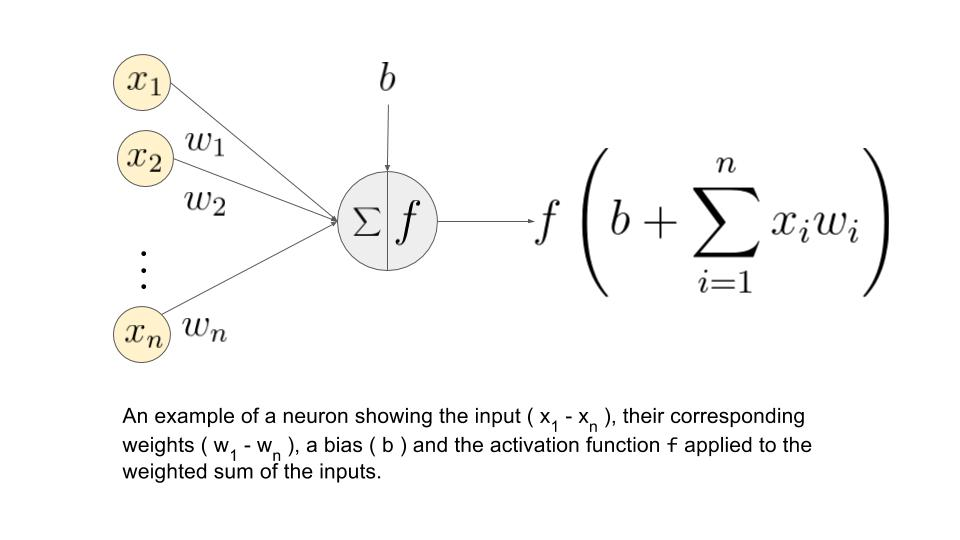# Convolutional Neural Networks¶

## What is image?¶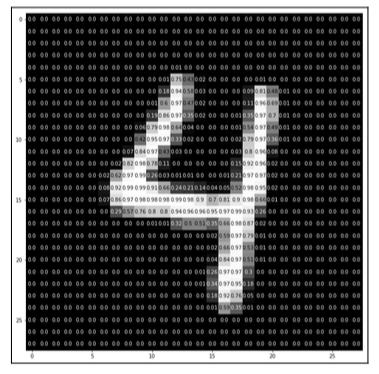• Multi-dimentional array
• Key characteristics:
• width
• height
• depth
• range
• Example
• RGB images have depth of 3 (one for each of R, G, B)
• Usually color intencity range is from 0 to 255 (8 bits)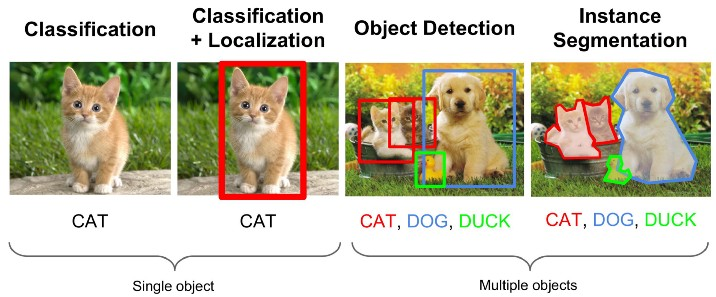# Computer Vision (CV)¶

• There were (are) many things before CNN Hype

# Threshold segmentation¶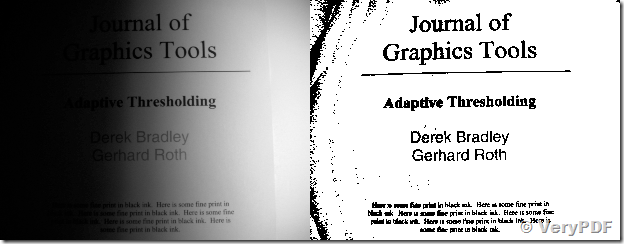## Threshold segmentation¶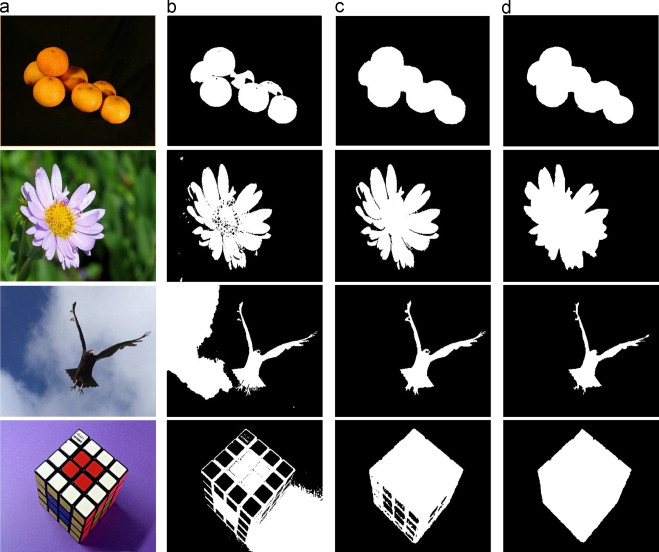## Template Matching¶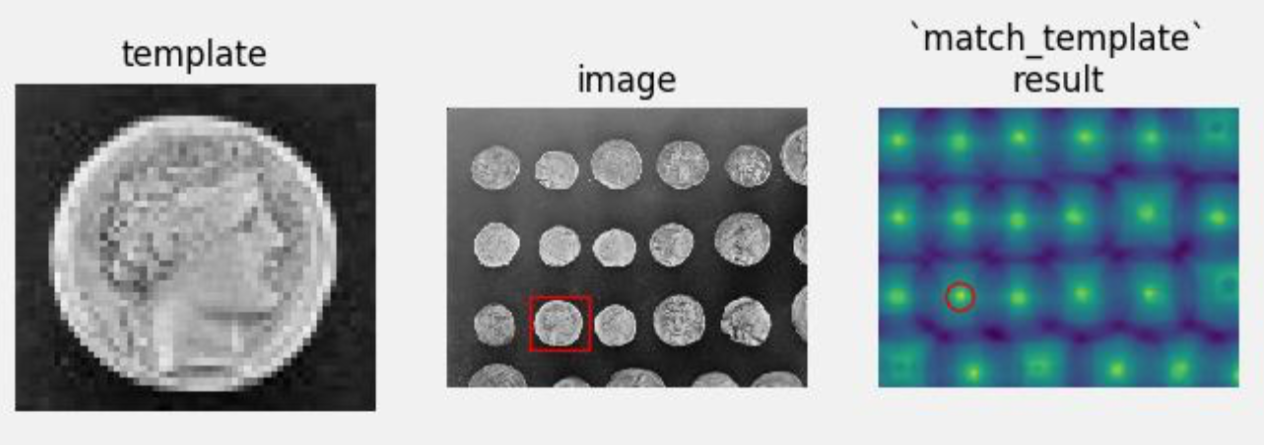## Object Detection with Viola-Jones and Haar¶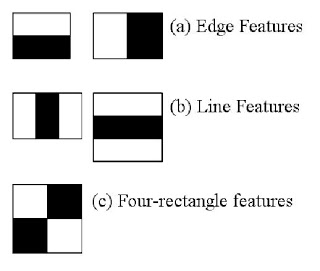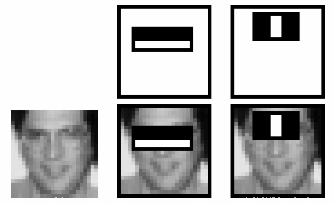## Object Detection with Viola-Jones and Haar¶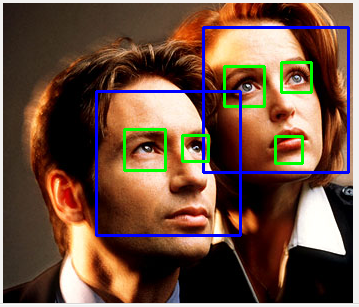## Edge Detection - Sobel Operator¶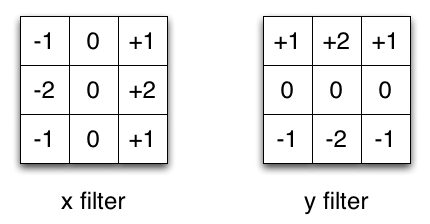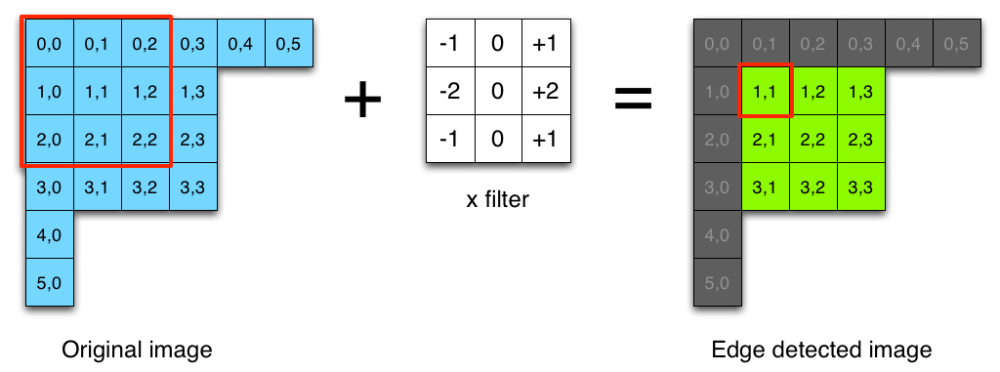## It is actually a convolution filter!¶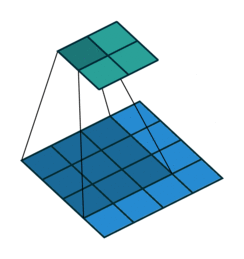## Result¶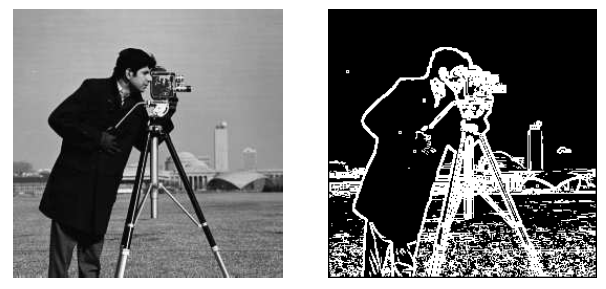# Conv Nets!¶

## Typical CNN Architecture¶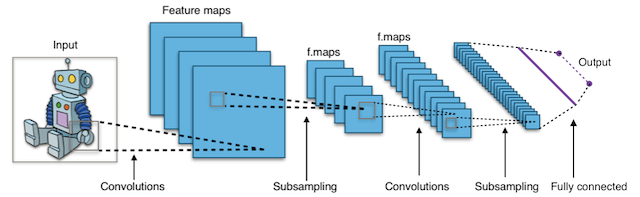## Convolution Filters¶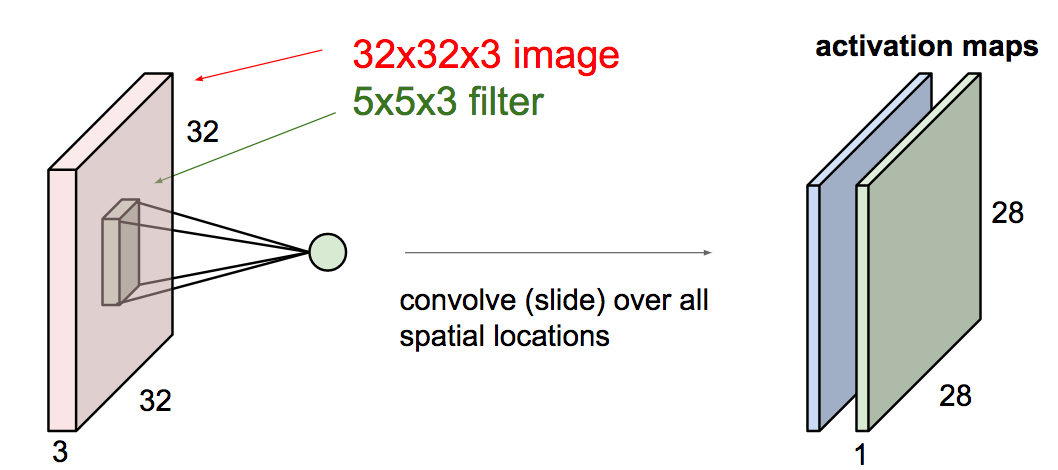>

(c) cs231n

## Convolution Filters¶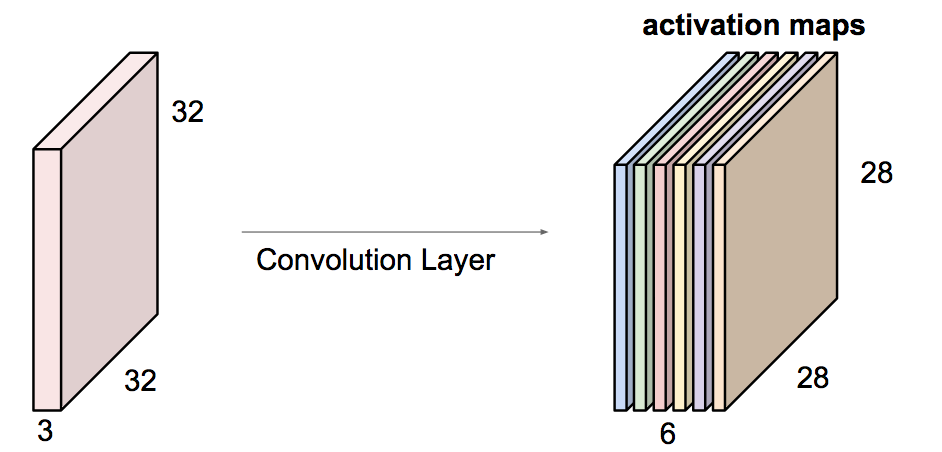(c) cs231n

## Convolution Filters¶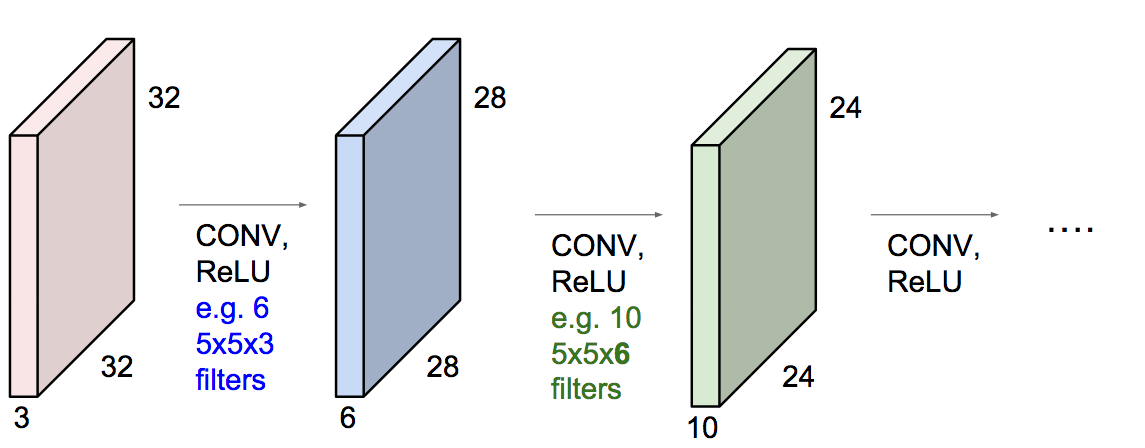(c) cs231n

## Features¶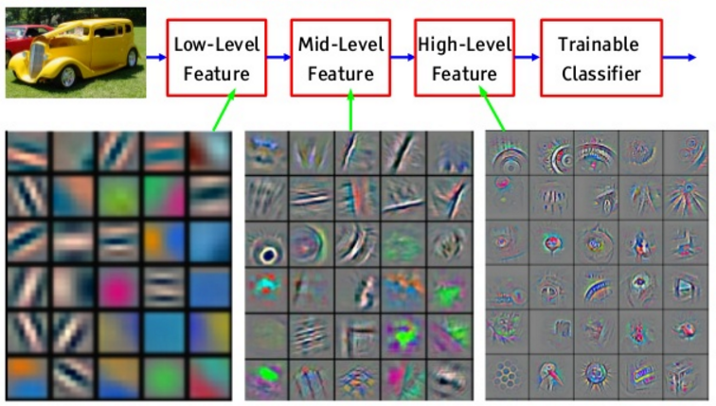## Key Specs of Conv Filters¶

• Number of filters
• Filter Size ($K$)
• Padding Size ($P$)
• Stride Size ($S$)

If number of input features is $N_{in}$ how to calculate number of output features $N_{out}$?

$$N_{out} = \frac{N_{in} + 2P - K}{S} + 1$$

## Number of parameters to learn?¶

• Input: $32 \times 32 \times 3$
• $20$ Filters: $5 \times 5$, $P = 1$, $S = 2$
• Number of weights?
$$20\times(5 \times 5 \times 3 + 1)$$

# Backprop through convolution¶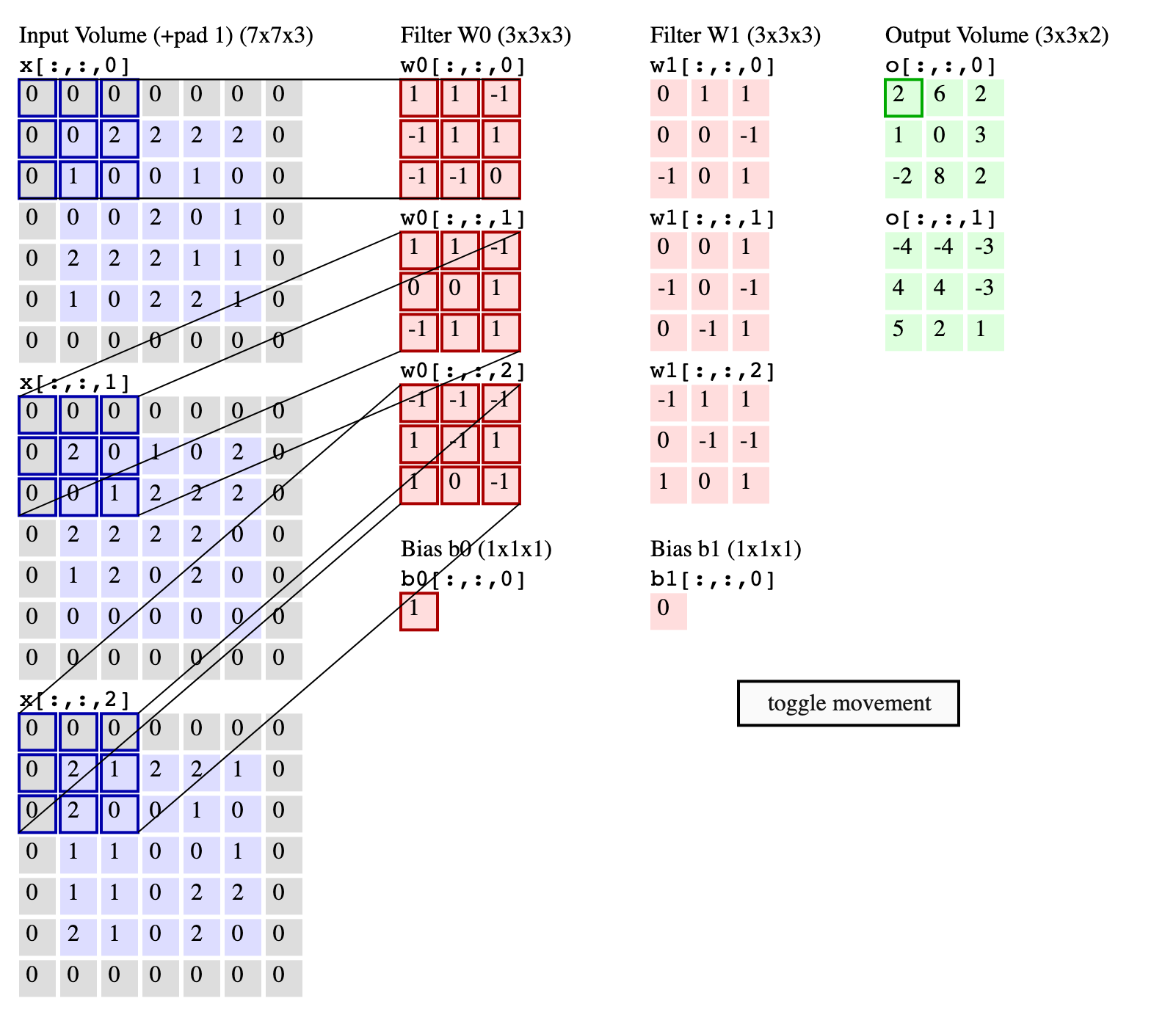(c) cs231n

Lets simplify things a bit: consider only a single channel $5 \times 5$ input and $3 \times 3$ convolutional filter.

No strides, no padding, no bias weight.

\begin{align} \left( \begin{array}{ccccc} 0 & 1 & 2 & 1 & 0 \\ 4 & 1 & 0 & 1 & 0 \\ 2 & 0 & 1 & 1 & 1 \\ 1 & 2 & 3 & 1 & 0 \\ 0 & 4 & 3 & 2 & 0 \\ \end{array} \right) &\quad * & \left( \begin{array}{ccc} 0 & 1 & 0 \\ 1 & 0 & 1 \\ 2 & 1 & 0 \\ \end{array} \right) & \quad = & \left( \begin{array}{ccc} 9 & 5 & 4 \\ 8 & 8 & 10 \\ 8 & 15 & 12 \\ \end{array} \right) \\ \mathbf{X} \qquad \qquad &\quad * & \mathbf{W} \qquad & \quad = & \mathbf{I} \qquad \end{align}

Denote

• $I_{i,j}$ - input $(i,j)$-th element of feature map ("neuron")
• $O_{i,j}$ - "activated" $(i,j)$-th element of feature map ("neuron")

Go down to indices (filter indexing starts from "center"):

$$I_{i,j} = \sum\limits_{-1 \leq a,b \leq 1} W_{a,b}X_{i+a, j+b}$$

And usually some activation function $f(\cdot)$ is applied: $$O_{i,j} = f(I_{i,j})$$

Similarly to backprop algorithm in previous lecture:

• Define $\delta_{i,j}$ as error in $(i,j)$ position of feature map: $$\delta_{i,j} = \frac{\partial L}{\partial I_{i,j}} = \frac{\partial L}{\partial O_{i,j}}\frac{\partial O_{i,j}}{\partial I_{i,j}} = \frac{\partial L}{\partial O_{i,j}} f'(I_{i,j})$$
• To compute $\frac{\partial L}{\partial W_{a,b}}$ we have to take into account that weight as "shared" $$\frac{\partial L}{\partial W_{a,b}} = \sum_i \sum_j \frac{\partial L}{\partial I_{i,j}} \frac{\partial I_{i,j}}{\partial W_{a,b}} = \sum_i \sum_j \delta_{i,j} X_{i+a, j+b}$$
• Finally, to pass gradients to previous layers: $$\frac{\partial L}{\partial X_{i,j}} = \sum_{a,b} \frac{\partial L}{\partial I_{i-a, j-b}} \frac{\partial I_{i-a, j-b}}{\partial X_{i, j}} = \sum_{a,b} \delta_{i-a,j-b} W_{a,b}$$

OMG, it is convolution too!

## Pooling layers¶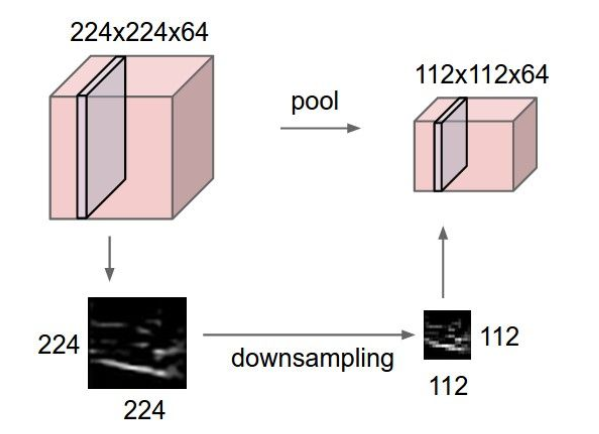## Pooling layers¶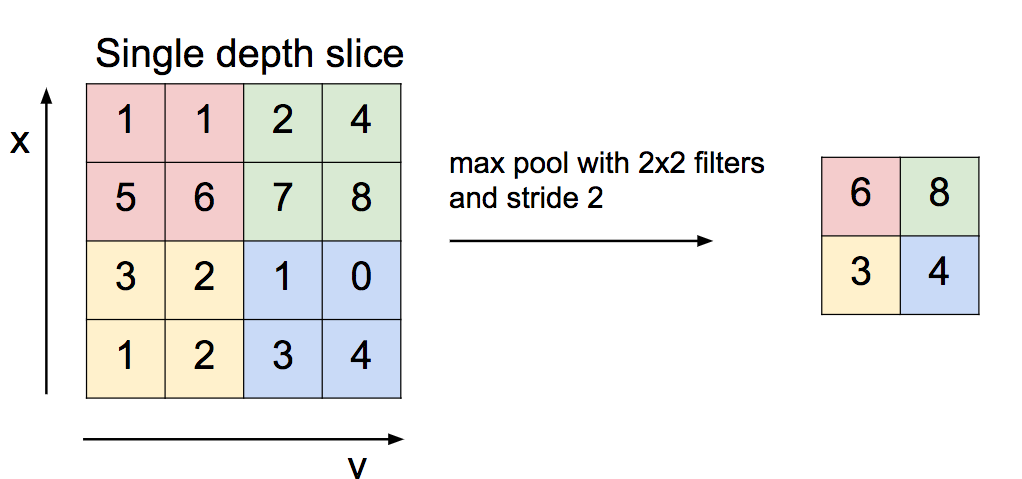What's with derivative here?

# Common Architectures¶

## AlexNet¶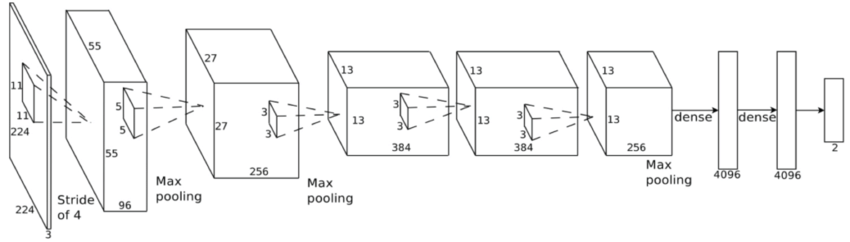• Max-pooling
• Re-LU activation
• 60M weights
• First place in the 2012 ImageNet competition
• ConvNets hype begins!
• Paper

## LeNet5 (1998)¶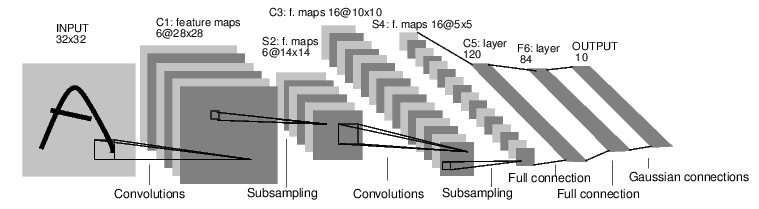• Hand-written characters recognition
• Avg-Pooling
• 60 000 weights
• Paper

## VGG 16¶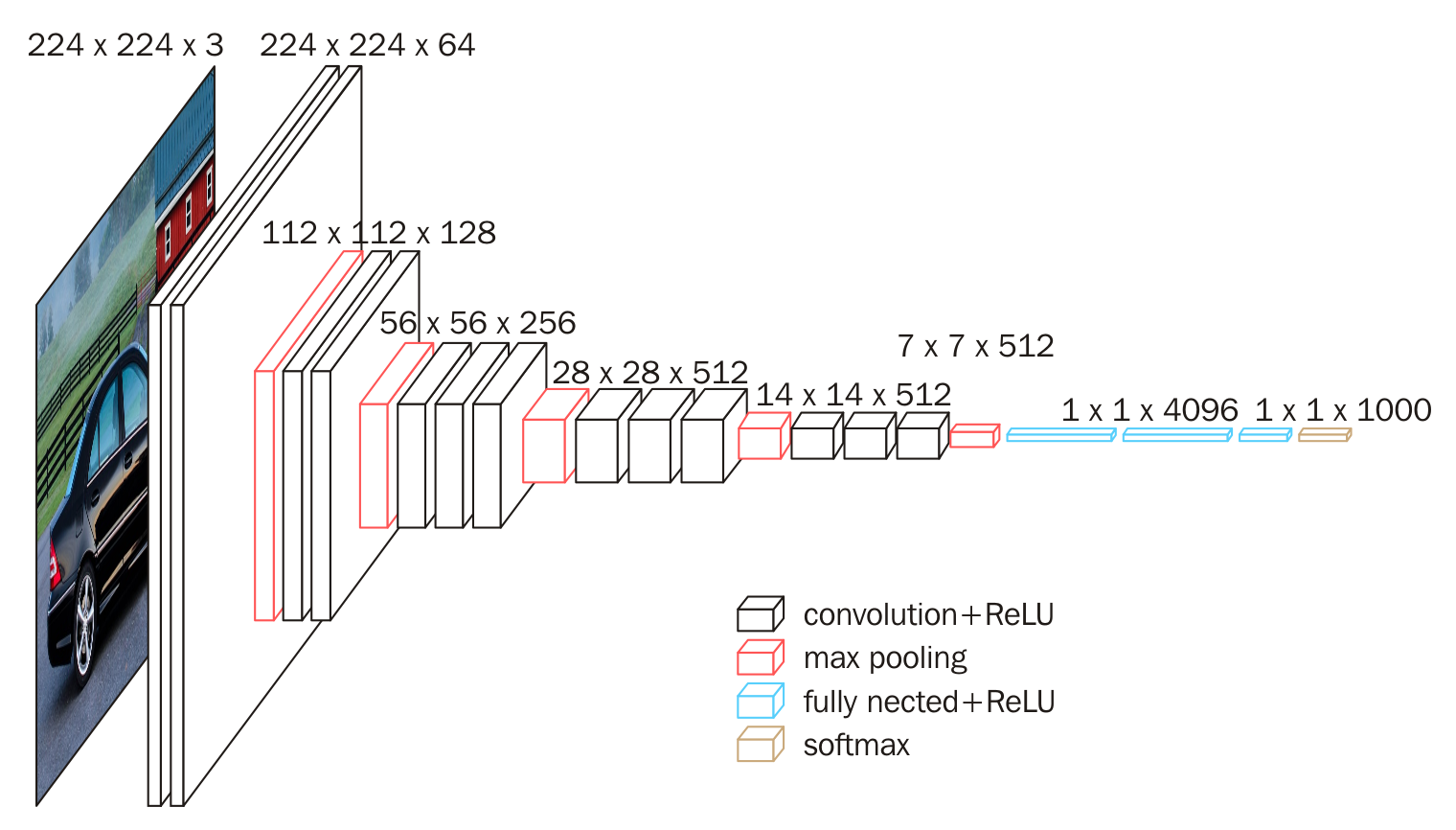• 140 M parameters
• Freezing weights during learning
• Paper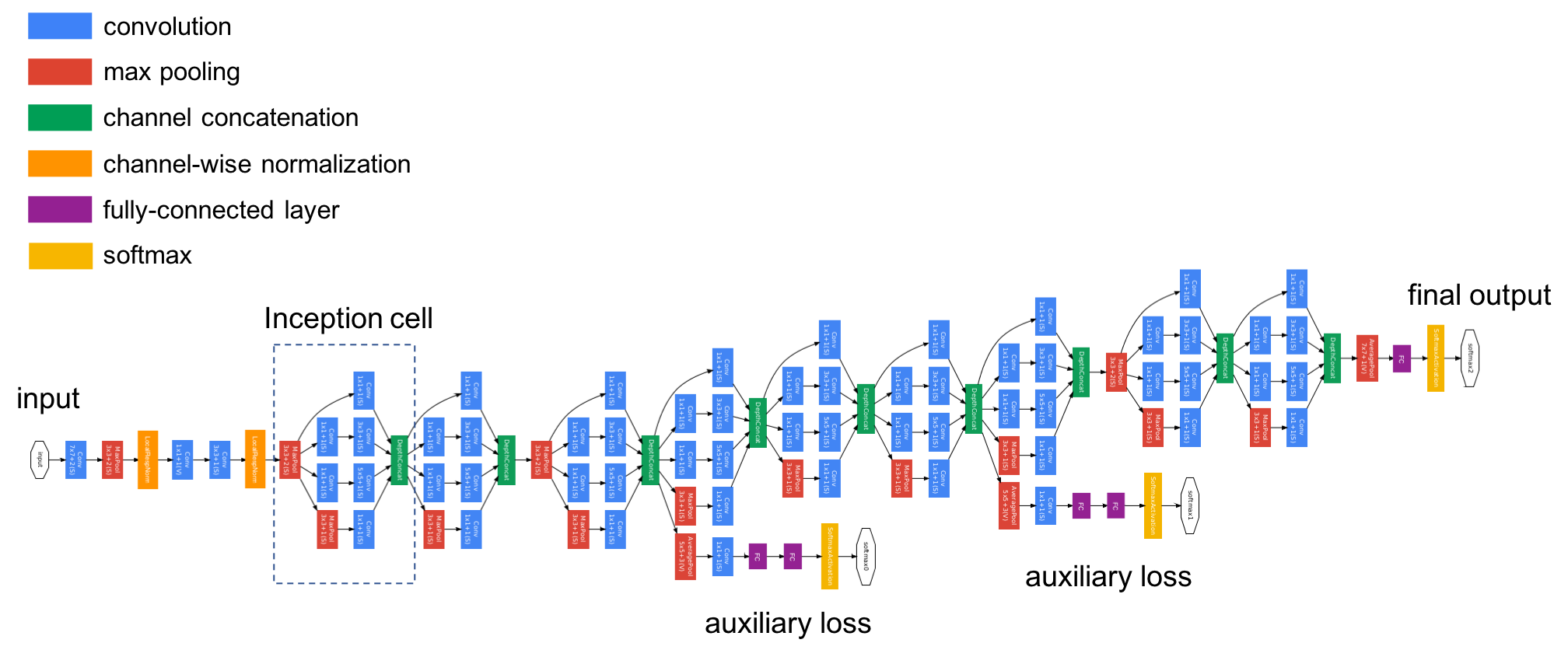• 5 (V1) parameters
• Introduction of batch normalization and residual blocks
• Paper

## ResNet¶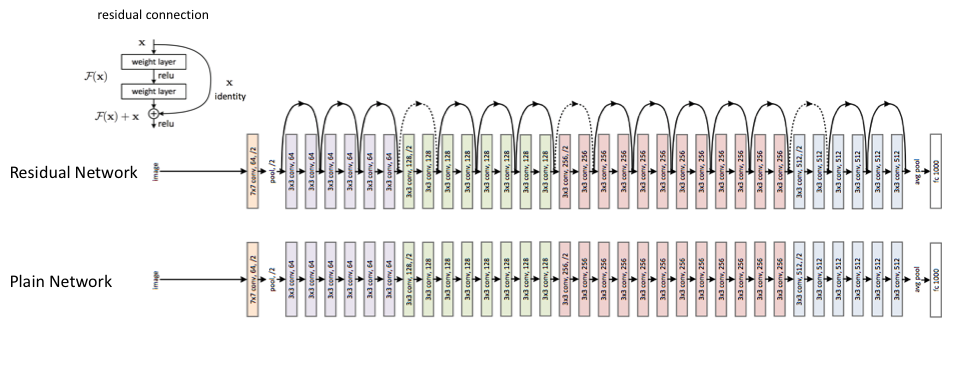• ULTRA DEEP
• Residual connections
• Paper

## ConvNets for text¶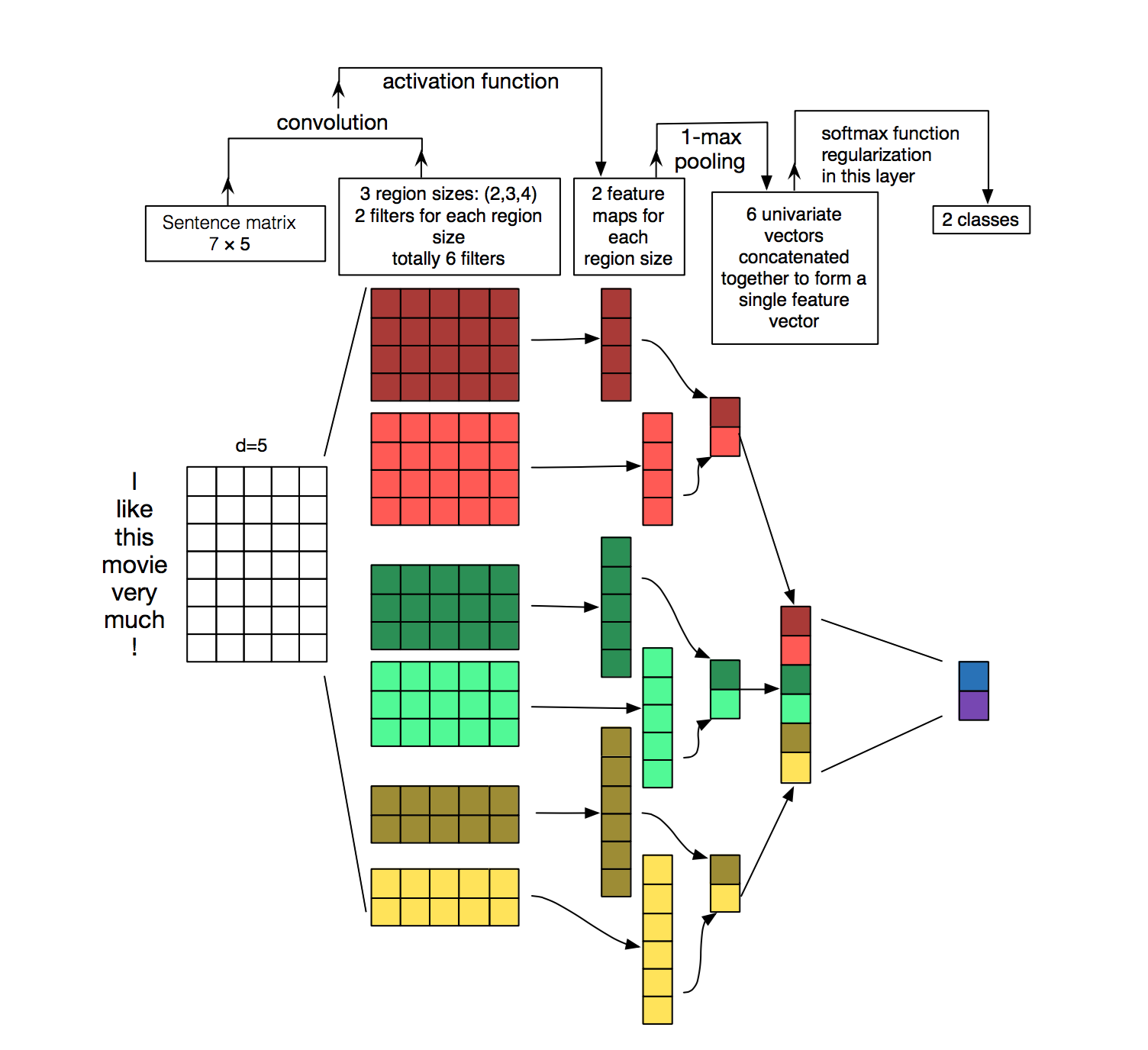## Autoencoders¶

• Autoencoders tries to recover input signal from compressed representation
• so they should save only the most valuable inforation!
• dimention reduction!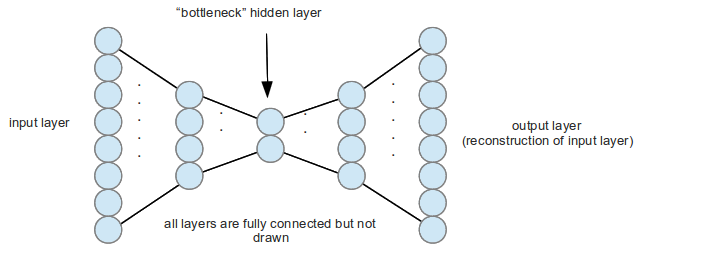## Autoencoders¶